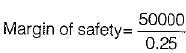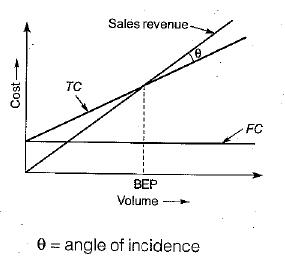Courses

# Test: Break Even Analysis - 1

## 10 Questions MCQ Test Topicwise Question Bank for Mechanical Engineering | Test: Break Even Analysis - 1

Description
This mock test of Test: Break Even Analysis - 1 for Mechanical Engineering helps you for every Mechanical Engineering entrance exam. This contains 10 Multiple Choice Questions for Mechanical Engineering Test: Break Even Analysis - 1 (mcq) to study with solutions a complete question bank. The solved questions answers in this Test: Break Even Analysis - 1 quiz give you a good mix of easy questions and tough questions. Mechanical Engineering students definitely take this Test: Break Even Analysis - 1 exercise for a better result in the exam. You can find other Test: Break Even Analysis - 1 extra questions, long questions & short questions for Mechanical Engineering on EduRev as well by searching above.
QUESTION: 1

### A spare parts retail shop has sales of Rs. 400,000 and a profit of Rs. 50,000 for a product, in its first quarter. The profit volume (PV) ratio is 25%. The margin of safety = profit/PV ratio. The break even point of sales (in ...... Rs.) is

Solution:= Rs. 2,00,000
Margin of safety = Present sales - Break - even-sales
∴ Break-even-sales = 4,00,000-2,00,000
= 2,00,000

QUESTION: 2

Solution:
QUESTION: 3

### Berak-even analysis is associated with

Solution:
QUESTION: 4

For break-even analysis, the cost takes into account

Solution:
QUESTION: 5

Break-even point is the point where

Solution:QUESTION: 6

Angle of incidence is the included angle between income-line and ........ at the break even point in a break-even chart

Solution:
QUESTION: 7

If the fixed cost of the assets for a given period quadruples, then how much will the break-even quantity become?

Solution:
QUESTION: 8

Breakeven analysis consists of

Solution:
QUESTION: 9

In breakeven analysis, total cost consist of

Solution:
QUESTION: 10

Process X has a fixed cost of Rs. 50,000 per month and a variable cost of Rs. 10 per unit. Process Y has a fixed cost of Rs. 20,000 per month and a variable cost of Rs. 25 unit. At which value, total costs of processes X and Y will be equal

Solution:

TCX ⇒ TCY
⇒ 50,000 + 10 N = 20,000 + 25 N
∴ 15 N = 30,000
∴ N = 2,000 unit